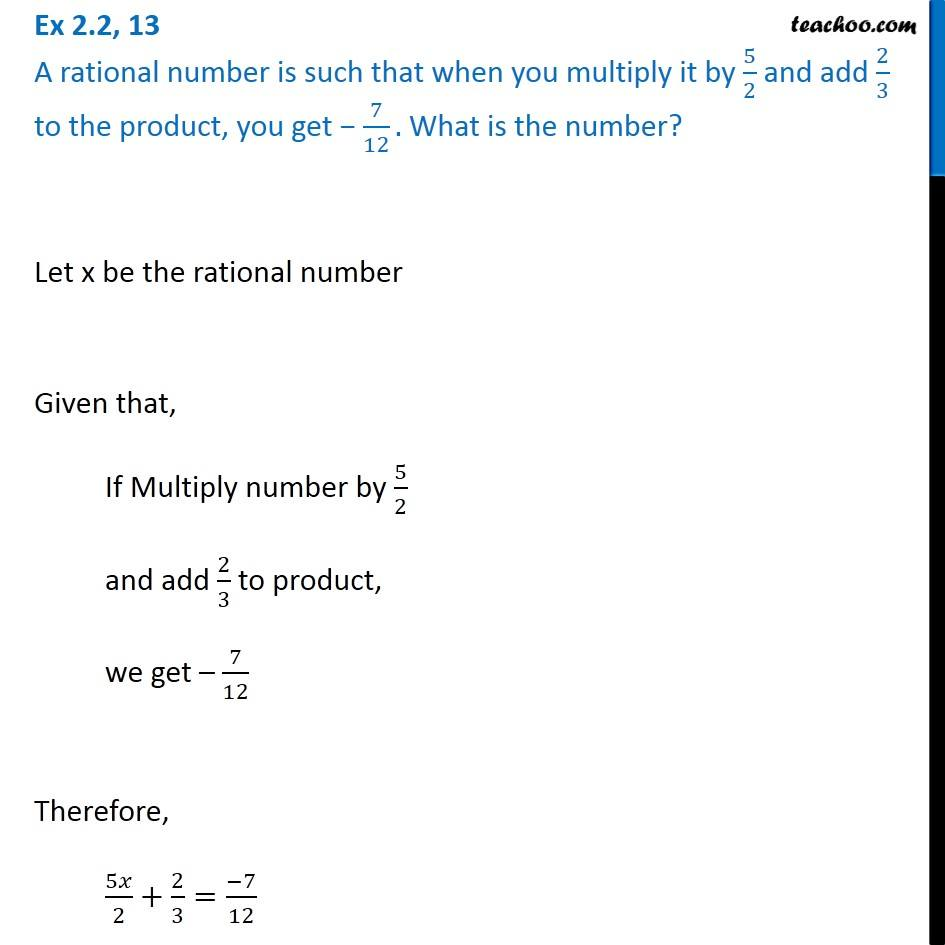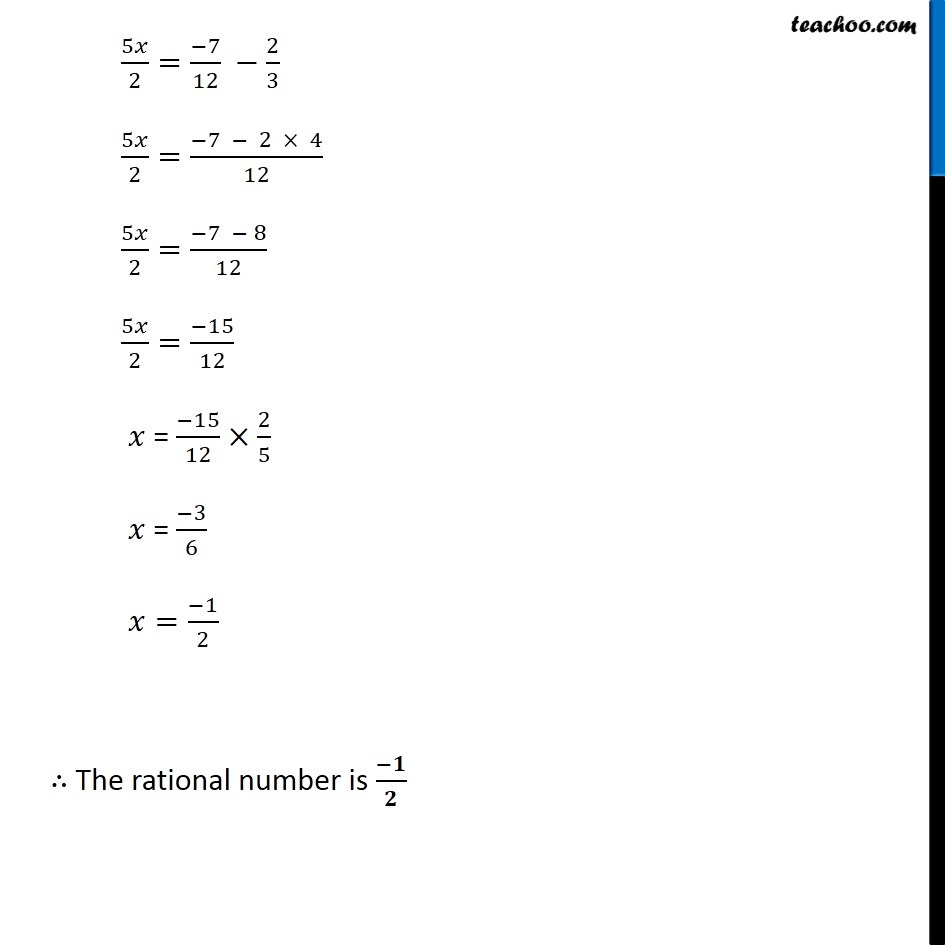Ex 2.2

Chapter 2 Class 8 Linear Equations in One Variable
Serial order wiseGet live Maths 1-on-1 Classs - Class 6 to 12

### Transcript

Ex 2.2, 13 A rational number is such that when you multiply it by 5/2 and add 2/3 to the product, you get − 7/12. What is the number?Let x be the rational number Given that, If Multiply number by 5/2 and add 2/3 to product, we get – 7/12 Therefore, 5𝑥/2+2/3=(−7)/12 5𝑥/2=(−7)/12 −2/3 5𝑥/2=(−7 − 2 × 4)/12 5𝑥/2=(−7 − 8)/12 5𝑥/2=(−15)/12 𝑥 = (−15)/12×2/5 𝑥 = (−3)/6 𝑥=(−1)/2 ∴ The rational number is (−𝟏)/𝟐